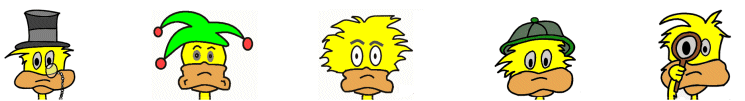# Kids Math

## Glossary and Terms: Measurement

Area - The measurement of the surface of an area is defined in square units. The area is the number of squares that fit inside the region. The formula for determining the area of a rectangle is length x width.

Example: Area = 5 x 6 = 30 square units, where the length = 5 units and the width = 6 units

Capacity - The capacity is the measurement of how much something will hold. It is also the volume of an object.

Cubed - Cubed is a three dimensional measurement of a cube with all three sides the same size. It is represented by a small 3 next to the number like X³.

Example: 5 cubed = 5³ = 5 x 5 x 5 = 125

Degree - A unit of measure used for angles.

Example: A circle is divided up into 360 degrees. A right angle is 90 degrees.

Dimension - In measurement, the dimensions are represented by length, width, and height.

Distance - The length between two points as drawn by a straight line.

Example: It is 5 miles from my house to my school. The distance is 5 miles.

Estimate - When guessing a measurement close to the exact measurement, this is called an estimate.

Height - The measurement of the vertical distance.

Example: That girl is 5 feet tall. Her height is 5 feet.

Length - A measurement of distance. It is usually the longer of two distances of an object, the other being the width.

Example: A football field is 100 yards long. Its length is 100 yards.

Measurement - Determining the physical quantity of something such as length, time, temperature, or volume in terms of a unit of measurement such as feet, seconds, or degrees.

Perimeter - The perimeter is the path that surrounds an area. The distance of the path is the measurement of the perimeter.

Example: The perimeter of this polygon is 3 + 7 + 5 + 4 + 6 + 4 = 29.Rounding - Rounding a number or measurement is when you write the number in a shorter or simpler form that is an approximation of the exact number or measurement.

Example:
671.98 rounded to the nearest 1s place is 672.
5,213 rounded to the nearest 100s place is 5,200.

Ruler - A ruler is a tool used to measure distance. It can also help to draw straight lines.

Surface Area - The total surface area of three dimensional object is the added areas of all the objects surfaces (for a cube this would be 6 sides).

Example: The total surface area of a cube with side length 4 would be 4x4 = 16 (for each side). Then multiply by 6 for the six sides: 16x6 = 96.

Temperature - The measurement of an object that expresses its hotness or coldness. The units of measurement are degrees Fahrenheit or degrees Celsius.

Example: The oven was hot, it was 350 degrees F.

Thermometer - A device for measuring temperature.

Time - A measurement of the dimension that represents intervals of the past, present, and future. Units of time include seconds, minutes, and hours.

Unit - A unit of measurement is a standard by which measurements can be compared. Different types of measurements use different units.

Example: Inches are a unit of measurement used to measure distance. Other units of measurement include seconds (time), degrees (temperature), and grams (mass).

Volume - The quantity of space that an object takes up. It is measured in cubic units.

Example: The volume of a box is equal to the length x width x height.

Weight - The measurement of the pull of gravity on an object. Typical units include pounds, ounces, and tons.

Width - The measurement of the distance of a side of an object. Usually this is the shorter side while the length is the longer side.

More Math Glossaries and Terms

Algebra glossary
Angles glossary
Figures and Shapes glossary
Fractions glossary
Graphs and lines glossary
Measurements glossary
Mathematical operations glossary
Probability and statistics glossary
Types of numbers glossary
Units of measurements glossary

Back to Kids Math

Back to Kids Study